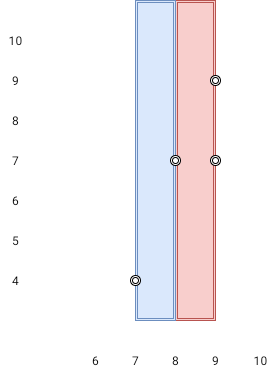##### Welcome to Subscribe On Youtube

Formatted question description: https://leetcode.ca/all/1637.html

# 1637. Widest Vertical Area Between Two Points Containing No Points (Medium)

Given n points on a 2D plane where points[i] = [xi, yi], Return the widest vertical area between two points such that no points are inside the area.

A vertical area is an area of fixed-width extending infinitely along the y-axis (i.e., infinite height). The widest vertical area is the one with the maximum width.

Note that points on the edge of a vertical area are not considered included in the area.

Example 1:Input: points = [[8,7],[9,9],[7,4],[9,7]]
Output: 1
Explanation: Both the red and the blue area are optimal.


Example 2:

Input: points = [[3,1],[9,0],[1,0],[1,4],[5,3],[8,8]]
Output: 3


Constraints:

• n == points.length
• 2 <= n <= 105
• points[i].length == 2
• 0 <= xi, yi <= 109

Related Topics:
Sort

## Solution 1. Sort

Sort the points in ascending order of x values, then traverse to find the maximum distance of x values between adjacent points.

// OJ: https://leetcode.com/problems/widest-vertical-area-between-two-points-containing-no-points/
// Time: O(NlogN)
// Space: O(1)
class Solution {
public:
int maxWidthOfVerticalArea(vector<vector<int>>& A) {
sort(begin(A), end(A), [](auto &a, auto &b) { return a< b; });
int ans = 0;
for (int i = 1; i < A.size(); ++i) ans = max(ans, A[i] - A[i - 1]);
return ans;
}
};


Java

• class Solution {
public int maxWidthOfVerticalArea(int[][] points) {
Arrays.sort(points, new Comparator<int[]>() {
public int compare(int[] point1, int[] point2) {
if (point1 != point2)
return point1 - point2;
else
return point1 - point2;
}
});
int maxWidth = 0;
int length = points.length;
for (int i = 1; i < length; i++) {
int width = points[i] - points[i - 1];
maxWidth = Math.max(maxWidth, width);
}
return maxWidth;
}
}

• // OJ: https://leetcode.com/problems/widest-vertical-area-between-two-points-containing-no-points/
// Time: O(NlogN)
// Space: O(1)
class Solution {
public:
int maxWidthOfVerticalArea(vector<vector<int>>& A) {
sort(begin(A), end(A), [](auto &a, auto &b) { return a< b; });
int ans = 0;
for (int i = 1; i < A.size(); ++i) ans = max(ans, A[i] - A[i - 1]);
return ans;
}
};

• class Solution:
def maxWidthOfVerticalArea(self, points: List[List[int]]) -> int:
points.sort()
return max(b - a for a, b in pairwise(points))

############

# 1637. Widest Vertical Area Between Two Points Containing No Points
# https://leetcode.com/problems/widest-vertical-area-between-two-points-containing-no-points/

class Solution:
def maxWidthOfVerticalArea(self, points: List[List[int]]) -> int:
if not points: return 0

points.sort(key = lambda x : (x, x))

res = 0
prevX = points
for x,y in points[1:]:
if x == prevX:
continue
else:
res = max(res, x - prevX)
prevX = x

return res